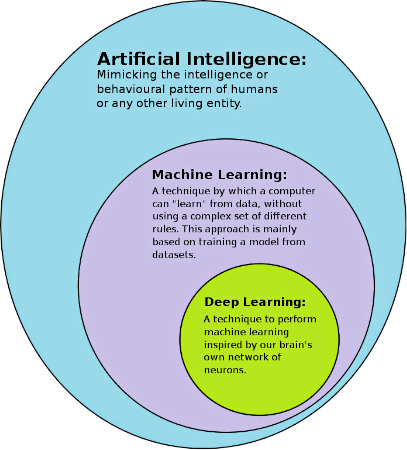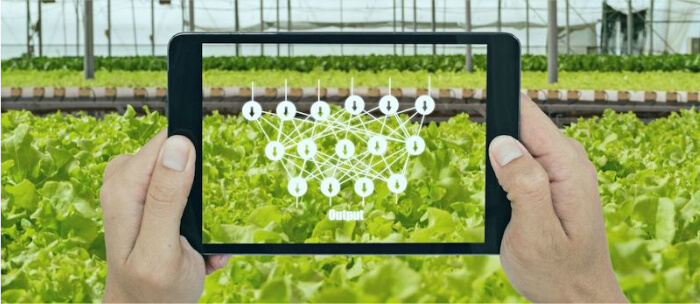Technical Article

Deep Learning vs. Machine Learning

November 26, 2021 by Anish Devasia

Deep learning is a subset of machine learning, which is a subset of artificial intelligence. So, what makes deep learning different from machine learning?

In the initial days of computers, scientists primarily used them to perform simple mathematical and logical operations. Then, computers slowly evolved to execute complex calculations, elaborate problem-solving, and form the information backbone of the world. Moving beyond the scope of traditional computing meant that the computer needed intelligence.

Researchers and computer engineers turned to mimic human intelligence. Artificial intelligence (AI) is the area of computer science dedicated to creating computing systems as intelligent as human beings. Initial AI models were computing systems that performed complex logical operations. Later, more advanced techniques were developed to perform smarter or “intelligent” tasks.

Two of the most used terms in AI are machine learning and deep learning. This article looks at the origins of these two techniques, the commonalities between them, and the differences.

Deep Learning and Machine Learning

Machine learning and deep learning are subsets of AI. Between them, deep learning is the subset of machine learning. This means all deep learning is machine learning but not all machine learning is deep learning. To understand the differences between them, the term machine learning excludes deep learning for the rest of this article.Machine Learning

Machine learning analyzes a large amount of data to understand the characteristics of a  training dataset. What has been learned from the training dataset is applied to other datasets to make “intelligent” decisions. The models created with the training dataset can work with other similar datasets to generate the desired output.

The training dataset for machine learning models should be labeled, and the developers must supervise and adjust the learning process as the new model is being trained. In addition to basic computing, statistical models are heavily used for training machine learning models.

Distinguishing between cats and dogs is a common example in the artificial learning space. Following is an overview of how machine learning models are trained with data.

The training model is fed with thousands of images of cats and dogs. Each of those images is labeled either “cat” or “dog.” The model in training identifies characteristics from the images that distinguish cats, dogs, and the rest of the objects. The traits are identified from the images using statistical techniques. Once the model has been trained with sufficient data, it is fed with unlabelled images. If the trained model can successfully distinguish between the images of cats and dogs with the desired accuracy, it is a successful machine learning model.

Deep Learning

Deep learning is the evolution of conventional machine learning. Humans do not learn with thousands of labeled examples; they learn automatically without much external help or validation. Deep learning takes machine learning a step closer to this model of intelligent learning.

Deep learning models must also be trained with a large volume of data, but models are not trained with labeled data. All the data fed to the deep learning model is unlabelled. The model identifies different elements from the data to give the required output.Figure 2. A visual representation of neural networks used in farming.

Deep learning models use complex mathematical systems called neural networks to learn from data. It contains multiple layers of mathematical functions with different weights. The “deep’” in the term deep learning is from these layers of processing.

Let us overview how deep learning models tackle distinguishing cats and dogs. A neural network is fed with numerous unlabeled images of cats and dogs. The neural network has to figure out there are two sets of animals in the pictures and then identify how to distinguish between the two animals. There is no labeled data required or supervision from developers.

Once a model is successfully trained, it can distinguish any number of images of cats and dogs.

Comparing Machine Learning and Deep Learning

The following table is a quick comparison of the differences between machine learning and deep learning. Keep in mind that deep learning is excluded from the term machine learning for this comparison, even though deep learning is a subset of machine learning.

 Machine Learning Deep Learning Training data Labeled data Unlabelled data Supervision Supervised learning Unsupervised learning Techniques Mostly statistical techniques Advanced mathematical functions Data volume Relatively less training data required A very large volume of data is required Accuracy Relatively low accuracy Higher accuracy with large data volume Training time Relatively less Very high training time

Industrial Applications for Deep Learning

Both machine learning and deep learning have industrial applications. Deep learning can be used in all applications that can be accomplished by machine learning. But deep learning requires more expertise, a much larger volume of data, higher computing power, and time. Due to these factors, the choice between both techniques involves consideration of a multitude of factors.

When higher accuracy is required, deep learning models are preferable. However, to achieve higher accuracy with deep learning models, a very large volume of data must be processed, thus involving a much longer training period. Machine learning algorithms can achieve routine usage in most instances.

Another general characteristic to consider is the complexity of the problem. As the complexity increases, deep learning models work better than machine learning models. However, there is no cut-and-dry rule on where to use the techniques. For example, machine learning is sufficient to analyze and predict the energy consumption in a plant. However, it is insufficient to build an automated quality control system—deep learning algorithms are needed in such scenarios.

Today, machine learning is much more accessible than deep learning, even in the industry. But in due course, deep learning models will improve, reducing implementation costs, and barriers to entry, meaning more computing power will be available at a lower price. The adoption of deep learning in the industry will rise over time.